# Magnetic Force as a Vector Product

The magnetic force exerted on a moving charge takes the form of a vector product.

You may enter values in any of the boxes below. Then click on the symbol for either the vector product or the angle.F= x10^N = x10^C( x10^ m/s)( T)(sin ) degrees.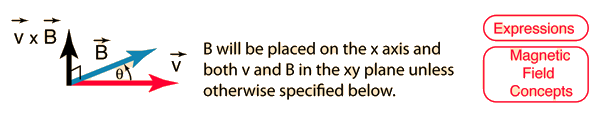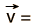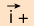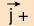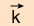x10^m/s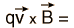x10^ Newtons magnitude.
Index

Vector concepts

Magnetic field concepts

 HyperPhysics***** Electricity and Magnetism R Nave
Go Back